## Questions

1. Given that A is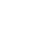and A-1 is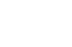Find the value of a and b in the expression: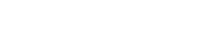(3 mks)
2. Solve for the unknowns given that the following is a singular matrix.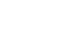3. Given that A =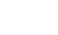and B =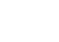and that C = AB, find C-1
4. B is a matrix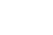and C is the matrix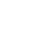If A is a 2 x 2 matrix and A x B = C. determine the matrix A.
5. An object of area 20 cm2 undergoes a transformation given by the matrix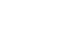followed by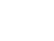find the area of the final image
6.  Find the matrix B such that AB = I and A =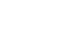Hence find the point of intersection of the lines 3x + 2y = 10 and 3y – 4 = x.
7. Given that P =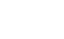and Q =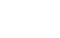find the matrix product of PQ. Hence solve the simultaneous eqution below
2x – 3y = 5
- x + 2y = – 3
8. Solve for x and y in the following matrix equation using elimination method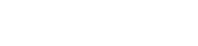9. A triangle XYZ , X (-1, -1) , Y (-2, -4) Z (-6 , -9) is reflected in the line X axis followed by a reflection in line X= Y. Find the image of the final image
10. Triangle ABC is the image of triangle PQR under a transformation M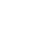where P, Q, R map onto A, B, C respectively.
1. Given the points P (5, -1) Q (6, -1) and R(4, - 0.5) draw the triangle ABC on the grid provided.
2. Triangle ABC in (a) above is to be enlarged by scale factor 2 with centre at (11, - 6) to map onto A1B1 and C1. Construct and label triangle A1B1 and C1 on the same grid.
3. By construction, find the coordinates of the centre and the angle of rotation which can be used to rotate triangle AIBICI onto triangle AIIBIICII whose coordinates are (-3, -2) , (-3, -6) and (-1, -2) respectively.
11. Triangle ABC with an area of 15 cm2 is mapped onto triangle AIBICI using matrix M =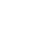. Find the area of triangle AIBICI.
12. T is a transformation represented by the matrix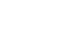under T a square whose area is 10cm2 is mapped onto a square of area 110cm2. Find the possible values of X
13. Triangle A1B1C1 is the image of ΔABC under a transformation represented by the matrix
M =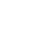If the area of triangle A1B1C1 is 54cm2. Determine the area of triangle ABC
14. Find the matrix B such that AB = I and A =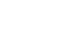. Hence find the point of intersection of the lines 3x + 2y = 10 and 3y – 4 = x

1.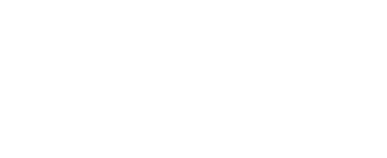2. (x-3) – (2x) = 0
x-3-2x = 0
-2x + x – 3 = 0
-x -3 = 0
x=3
3.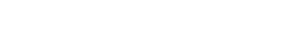Determinant = + 65 – 49 = 16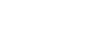4.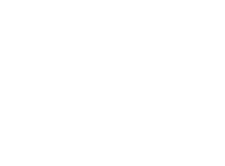5. 20x (-3 - -8)
100 area of 1
st image.
100 x (4 -
-3)
700 area of 2
nd image
6.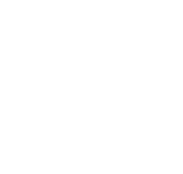7.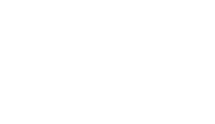8.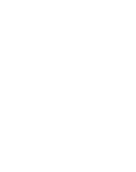9.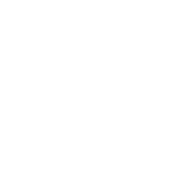10.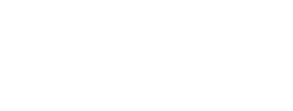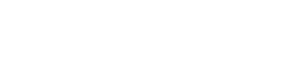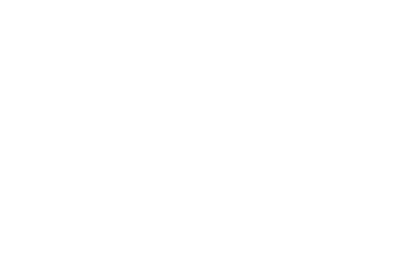11. Det 2 - -3 = 5
Area of A
IBICI = 5 x 15
= 75 cm
2
12. A.S.F = 110 = 11
10
5X (X) - -6 = 11
5X
2 + 6 = 11
5x
2 = 5
X
2 = 1
X = ±
1
13. Area of the image = Area of the object x Det.
Det. (
) = 15 – 18 = -3
54 cm
2 = A x -3
54 cm
2 = A
3
Area of
ABC = 18 cm2
14.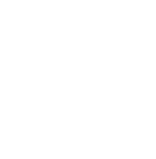#### Download Matrices Questions and Answers - Form 3 Topical Mathematics.

• ✔ To read offline at any time.
• ✔ To Print at your convenience
• ✔ Share Easily with Friends / Students

Read 7555 times Last modified on Wednesday, 10 February 2021 07:00
.
Subscribe now

access all the content at an affordable rate
or
Buy any individual paper or notes as a pdf via MPESA
and get it sent to you via WhatsApp